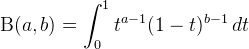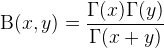Computes the Beta function.

Namespace:  Meta.Numerics.Functions
Assembly:  Meta.Numerics (in Meta.Numerics.dll) Version: 4.1.4Syntax
```public static double Beta(
double a,
double b
)```

#### Parameters

a
Type: SystemDouble
The first parameter, which must be positive.
b
Type: SystemDouble
The second parameter, which must be positive.

#### Return Value

Type: Double
The beta function B(a,b).Exceptions
ExceptionCondition
ArgumentOutOfRangeExceptiona or b is non-positive.Remarks

The Beta function can be defined by the integral:Equivalently, it can be defined as a commonly occurring ratio of Gamma functions:When evaluating such a ratio of Gamma functions, it is better to use this method than to call Gamma(Double) three times and form the ratio explicitly. One reason is that this method will be faster. Another reason is that, for many values, the individual Gamma functions will overflow even though the Beta function does not; this method will not overflow in such cases. There are still other cases in which the value of Beta does overflow or underflow a Double; in such cases, the method LogBeta(Double, Double) will still return an accurate value of its logarithm.

One place this ratio occurs is in the expression for a binomial coefficient in terms of factorials, so the Beta function can used to generalize binomial coefficients (BinomialCoefficient(Int32, Int32)) to non-integer values.See Also